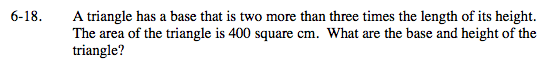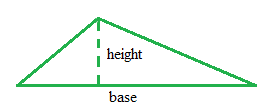### Home > MC2 > Chapter 6 > Lesson 6.1.2 > Problem6-18

6-18.Refer to problem 4-47 and use a method similar to the
5-D process to create an expression for the area of the triangle. Draw a diagram.

Based on the information from the problem, first define the height and base of the triangle with a variable.
Height: x
Base: 2 + 3x$\text{Since you know the area of a triangle, }$

$A = \frac{1}{2}\text{base(height),}$

$\text{construct a 5-D chart to run trials and solve for } x.\;\;\;\;\;\;\;\;\;\;\;$